Direct and Inverse Variation

Direct and Inverse Variation

Two variables vary directly if there is a nonzero number for which the following is correct: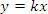In this equation, x and y are the two variables and k is the nonzero number, known as the constant of variation.

Graphs of Direct Variation

The graph of y = kx is a line through the origin, and the slope of said graph is k. From the graph we can see whether the value of k is positive or negative.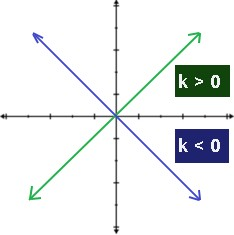Example

Find the constant of variation and the slope of the model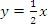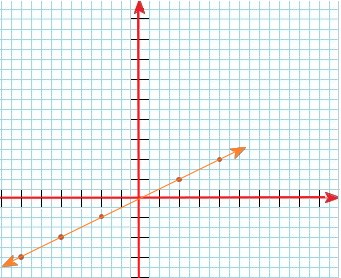For this equation, the constant of variation is k = 1/2. To find the slope, we use the slope formula: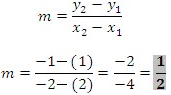We confirm with the answer that k is the slope of the graph.

Writing a Direct Variation Equation

If the variables x and y vary directly when x = 3 and y = 15, then:

a. Write an equation that relates x and y.

b. Find the value of y when x = 6.

Solution

a. Because x and y vary directly, the equation is in the form y = kx. We can then solve for k by using the given values for x and y.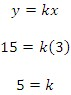The equation that relates x and y is y = 5x

b. To find the value of y, we substitute for x.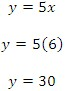Then when x = 6, y =30.

Using a Ratio to Model Direct Variation

The direct variation model can be rewritten in ratio form: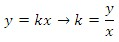The ratio form lets you know that when x and y have direct variation, the ratio of x to y is alike for all values of x and y. Occasionally, real-life figures can be approximated by a direct variation model, although the data might not fit the model exactly.

Example

The lengths (in centimeters) of 8 Maltese dogs at age 1 and age 10 are shown in the table below.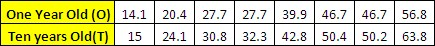a. Write a model that relates the length of the dogs at 1 year old to the length at 10 years old.

b. Estimate the body length of a Maltese whose length at 1 year was 26.40 cm.

Solution

a. We need to start by finding the ratio of 1 year to 10 years for each Maltese. Using the ratio form we obtain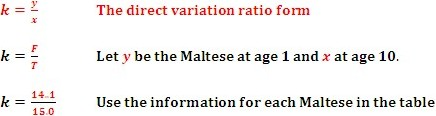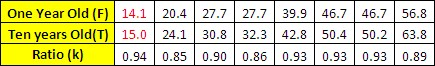b. Use the direct variation model to estimate the length of the Maltese.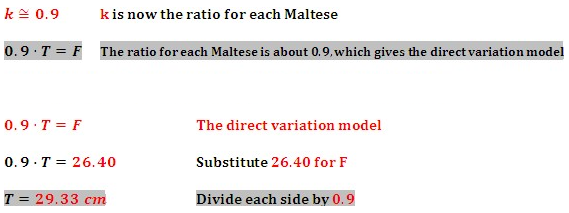Then we can estimate that the Maltese would be 29.33 cm at age 10.

Inverse Variation

For an inverse variation, k is still the constant of variation, but the model differs from direct variation. The variables x and y vary inversely for a constant k.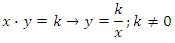Example

When x is 7 and y is 2, find an equation that inversely relates y and x.

Solution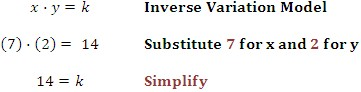The inverse variation that relates x and y is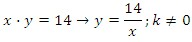Graphically it looks like this: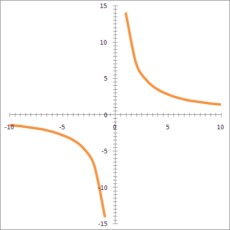Example

Compare the direct variation model and the inverse variation model for when x = 2 and y = 3.  Do this both numerically and graphically.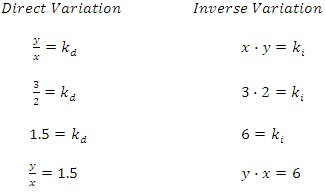a. Numerically:

• Direct Variation:  Because k is positive, y increases as x increases. So as x increases by 1, y increases by 1.5.
• Inverse Variation:  Because k is positive, y decreases as x increases.

We can create a table that will show the comparison: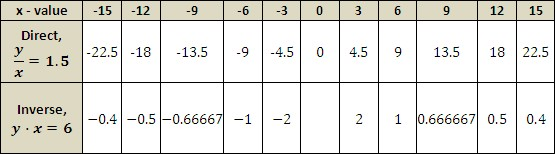b. Using the values on the table we can plot the points and then connect them to find the solution by graphing.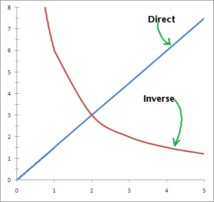• Direct Variation:  The graph for this model has the line passing through the origin with a positive slope.
• Inverse Variation:  The graph for this model is a curve that approaches the x-axis as x increases and approaches the y-axis as x gets closer to zero.